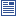Accessibility options:

# Differential Equations resources

Show me all resources applicable to

### Community Project (2)First Order Ordinary Differential Equations (SOURCE)
A zip file containing the LaTex source files and metatdata for the Teach Yourself resource First Order Differential Equations: A summary of five common methods to solve first order ODEs: direct integration, separation of variables, homogeneous equations, integrating factor and Bernouilli equations. This resource is contributed to the mathcentre Community Project by Morgiane Richard, University of Aberdeen and is reviewed by Shazia Ahmed, University of Glasgow.Second Order Ordinary Differential Equations (SOURCE)
A zip file containing the LaTeX source files and metadata for the Teach Yourself leaflet on Second Order Differential Equations: A summary of how to solve second order ODEs with constant coefficients: how to solve homogeneous equations (with no right hand-side) and how to solve equations with a right hand-side (method of undetermined coefficients and methods of variation of parameters). This Teach Yourself resource is contributed to the mathcentre Community Project by Morgiane Richard, University of Aberdeen and is reviewed by Shazia Ahmed, University of Glasgow.

### Teach Yourself (2)First Order Ordinary Differential Equations
First Order Differential Equations: A summary of five common methods to solve first order ODEs: direct integration, separation of variables, homogeneous equations, integrating factor and Bernouilli equations. This Teach Yourself resource is contributed to the mathcentre Community Project by Morgiane Richard, University of Aberdeen and is reviewed by Shazia Ahmed, University of Glasgow.Second Order Ordinary Differential Equations
Second Order Differential Equations: A summary of how to solve second order ODEs with constant coefficients: how to solve homogeneous equations (with no right hand-side) and how to solve equations with a right hand-side (method of undetermined coefficients and methods of variation of parameters). This Teach Yourself resource is contributed to the mathcentre Community Project by Morgiane Richard, University of Aberdeen and is reviewed by Shazia Ahmed, University of Glasgow.